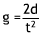# Physics

## Acceleration Due to Gravity

Measuring Tape
Red Bouncy Ball
Marble Ball
Baseball
0 1 2 3 4 5 6 7 8 9
0 1 2 3 4 5 6 7 8 9
:
0 1 2 3 4 5 6
0 1 2 3 4 5 6 7 8 9
:
0 1 2 3 4 5 6 7 8 9
0 1 2 3 4 5 6 7 8 9
Introduction
Materials
Instructions

Greek scientist and philosopher Aristotle (384–322 B.C.E) taught that heavier objects fall faster than lighter objects. His opinion was accepted as truth until the 15th century when Galileo Galilei, an Italian astronomer, physicist, and mathematician (1564–1642), challenged his ideas. Galileo performed experiments in which he dropped masses and measured the distance they traveled and the time it took them to fall. Later, he turned to inclined planes to study the effect of gravity on masses. He concluded that all objects traveling in the same medium will fall at a constant rate, regardless of their mass. Galileo’s experiments explained how objects move on Earth and how gravity affects their movements. His work laid the foundation for English physicist and mathematician Sir Isaac Newton (1643–1727), who developed the laws of motion. Galileo is remembered as the father of astronomy because of these ground-breaking ideas and for his improvements to the telescope. In this experiment, you will use both of Galileo’s methods to demonstrate acceleration due to gravity.

• bouncy ball
• measuring tape
• digital stopwatch
• inclined plane kit
• baseball
• marble
• data table

### Part A

1. Use a measuring tape to measure the height of the stand. You will use this distance measurement throughout the experiment.
2. From the top of the ramp, drop the bouncy ball to the ground. The stopwatch will automatically begin when you release the ball. Stop the stopwatch when the ball hits the ground. Record the time of the fall in the row labeled “Trial 1” under “Time (T)”of Data Table 1.
3. Calculate the acceleration of objects due to gravity (g) using the following formula:in which d represents distance and t equals time. Record your calculations for Trial 1 in the last column of Data Table 1.
4. Repeat steps 2 and 3 for two more trials.
5. Find the average for the three trials and record the average on Data Table 1.

### Part B

1. Place the marble at the top of the inclined plane and release it. The stopwatch will begin automatically.
2. Stop the stopwatch as soon as the marble reaches the bottom. Record the time on Data Table 2 in the row labeled “Trial 1.”
3. Repeat step 2 for two more trails with the marble.
4. Find the average for the three trials.
5. Calculate acceleration due to gravity using the formula from step 3 of Part A for each trial and the average from Data Table 2.
6. Repeat steps 2 through 6 using the baseball. Record your findings on Data Table 3.
Analysis
1. Did the marble and the baseball have the same, or nearly the same, acceleration due to gravity? How do you know?
2. The known value of acceleration due to gravity is 9.8 meters per second squared (m/sec2). Was your average for the acceleration due to gravity closer to the known value when you used the inclined plane or when you dropped the ball from the bleachers?
3. Why is it important to be precise when you measure time in this experiment?
4. Imagine that you have two pieces of notebook paper and you wad one into a ball. You drop both pieces from the same height at the same time. What happens?
5. Why do you think this happens if the acceleration due to gravity is the same for all objects?
1. Yes, because gravity acts on all objects in the same manner.